##### Actions

(diff) ← Older revision | Latest revision (diff) | Newer revision → (diff)

An algebraic equation of the second degree. The general form of a quadratic equation is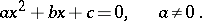In the field of complex numbers a quadratic equation has two solutions, expressed by radicals in the coefficients of the equation: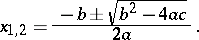(*)

When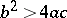both solutions are real and distinct, when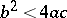, they are complex (complex-conjugate) numbers, when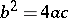the equation has the double root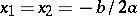.

For the reduced quadratic equation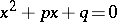formula (*) has the formThe roots and coefficients of a quadratic equation are related by (cf. Viète theorem):The expressionis called the discriminant of the equation. It is easily proved that, in accordance with the fact mentioned above that the equation has a double root if and only if. See also Discriminant. Formula (*) holds also if the coefficients belong to a field with characteristic different from 2.
Formula (*) follows from writing the left-hand side of the equation as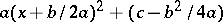(splitting of the square).